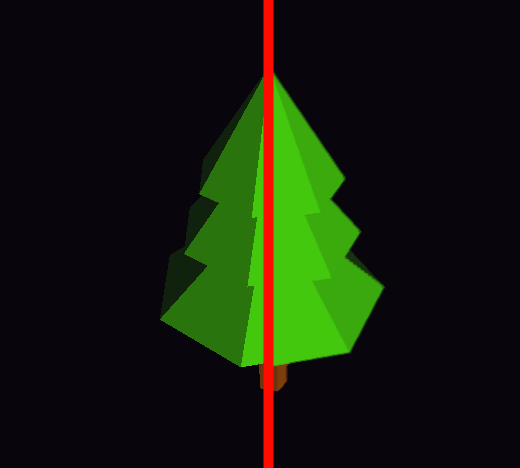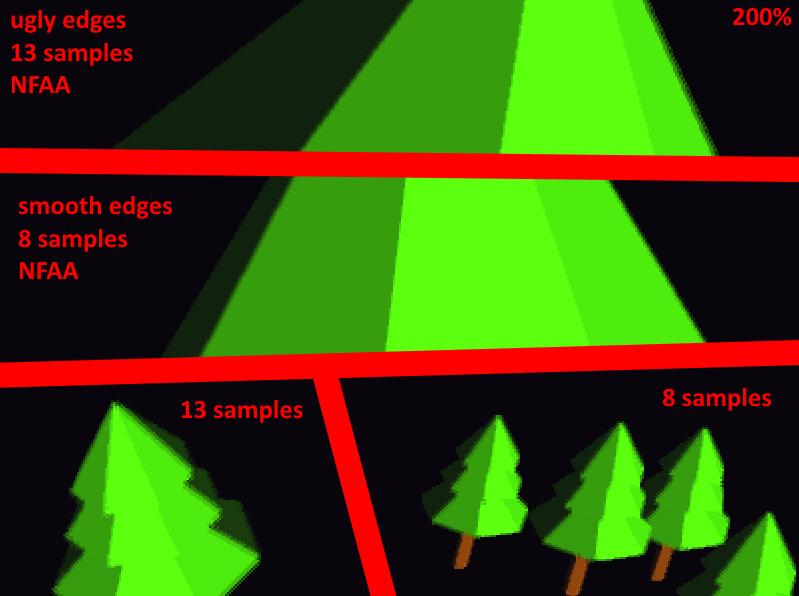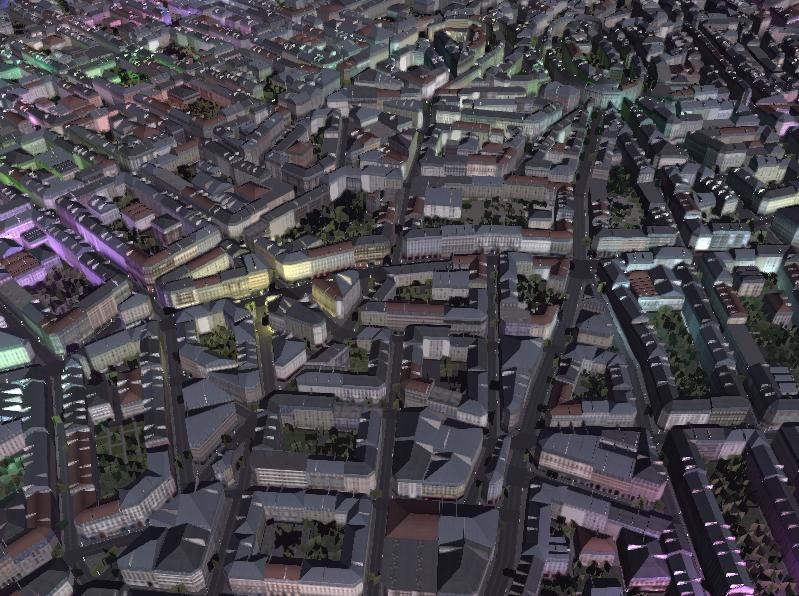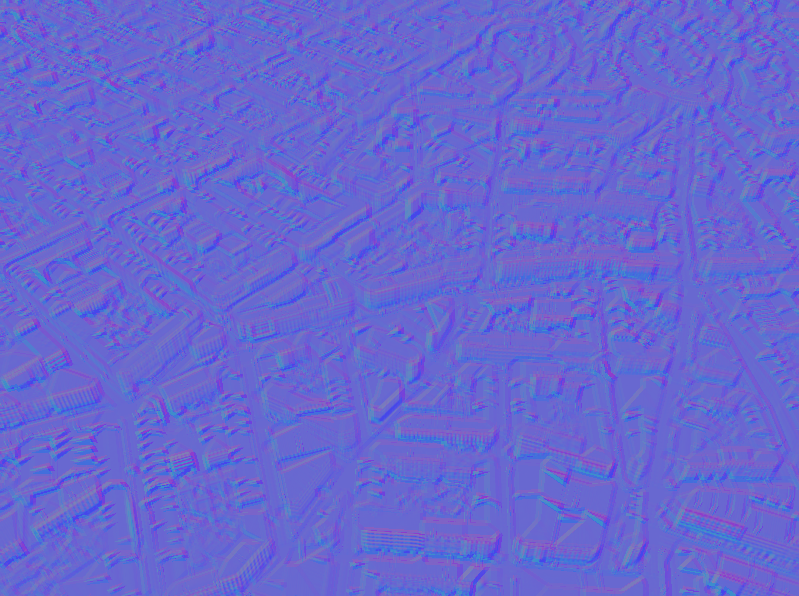Added post processing anti aliaising. Still tweaking it a bit.

The idea behind this shader: NFAA

Update:

Some improvements with less samples per pixel:And here is a rather high freqency image but with low color luminance varianc:And here is the same scene but with the edges detected:Update #2:

I went back to the 13 pixel lookups because testing only horizontal and vertical pixel differences caused on some “edge”-conditions no smoothing at all… Here’s my shader if anybody is interessted (Fragment shader):

```#version 400 //the final image buffer we want to smooth uniform sampler2D l_tex; uniform vec2 win_size;   //the output buffer layout( location = 0 ) out vec4 def_e;   //the spread of the edge detection algorithm const float fScale = 3.0f;   float GetColorLuminance( vec3 i_vColor ) { return dot( i_vColor, vec3( 0.2126f, 0.7152f, 0.0722f ) ); }   void main () {   vec2 st; st.s = gl_FragCoord.x / win_size.x; st.t = gl_FragCoord.y / win_size.y;   vec2 vPixelViewport = vec2( 1.0f / win_size.x, 1.0f / win_size.y );   // Offset coordinates vec2 upOffset = vec2( 0.0f , vPixelViewport.y ) * fScale; vec2 rightOffset = vec2( vPixelViewport.x, 0.0f ) * fScale;   float topHeight = GetColorLuminance( texture( l_tex, st + upOffset).rgb ); float bottomHeight = GetColorLuminance( texture( l_tex, st - upOffset).rgb ); float rightHeight = GetColorLuminance( texture( l_tex, st + rightOffset).rgb ); float leftHeight = GetColorLuminance( texture( l_tex, st - rightOffset).rgb );   float leftTopHeight = GetColorLuminance( texture( l_tex, st - rightOffset + upOffset).rgb ); float leftBottomHeight = GetColorLuminance( texture( l_tex, st - rightOffset - upOffset).rgb ); float rightBottomHeight = GetColorLuminance( texture( l_tex, st + rightOffset + upOffset).rgb ); float rightTopHeight = GetColorLuminance( texture( l_tex, st + rightOffset - upOffset).rgb );   // Normal map creation float sum0 = rightTopHeight+ topHeight + rightBottomHeight; float sum1 = leftTopHeight + bottomHeight + leftBottomHeight; float sum2 = leftTopHeight + leftHeight + rightTopHeight; float sum3 = leftBottomHeight + rightHeight + rightBottomHeight;   float vector1 = (sum1 - sum0); float vector2 = (sum2 - sum3);   //for horizontal and vertical only... //float vector1 = -(topHeight - bottomHeight); //float vector2 = rightHeight - leftHeight;   vec3 Scene0 = texture( l_tex, st ).rgb;   if(abs(vector1) &lt; 0.0001 || abs(vector2) &lt; 0.0001){ //we don't need to smooth ... def_e.rgb = Scene0; } else{ // Put them together and scale. vec2 Normal = vec2( vector2, vector1); Normal.xy = clamp(Normal,vec2(-1.0,-1.0)*0.4,vec2(1.0,1.0)*0.4); //Prevent over-blurring in high-contrast areas! -venzon vec2 final_norm = Normal * vPixelViewport * fScale; // Increase pixel size to get more blur   vec3 Scene1 = texture( l_tex, st + final_norm.xy ).rgb; vec3 Scene2 = texture( l_tex, st - final_norm.xy ).rgb; vec3 Scene3 = texture( l_tex, st + vec2(final_norm.x, -final_norm.y) * 0.5f ).rgb; vec3 Scene4 = texture( l_tex, st - vec2(final_norm.x, -final_norm.y) * 0.5f ).rgb;   //Final color smoothed def_e.rgb = (Scene0 + Scene1 + Scene2 + Scene3 + Scene4) * 0.2; //show the normals of the smooth pixels //def_e.rgb = normalize( vec3(Normal.x, Normal.y , 1.0f ) * 0.5f + 0.5f); //show pixels where the smoothing is applied to //def_e.rgb = vec3(1.0,0.0,0.0); } def_e.a = 1.0f; }```